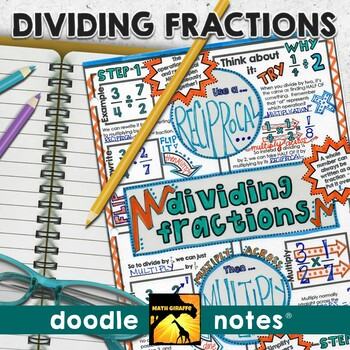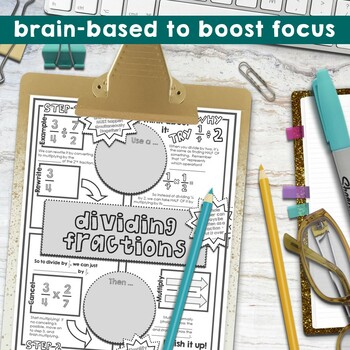# Dividing Fractions Doodle Notes5th - 7th
Subjects
Standards
Resource Type
Formats Included
• PDF
Pages
3 plus answer keys & info

### Description

Fraction Division: "doodle notes" -

When students color or doodle in math class, it activates both hemispheres of the brain at the same time. There are proven benefits of this cross-lateral brain activity:

- new learning

- relaxation (less math anxiety)

- visual connections

- better memory & retention of the content!

Students fill in the sheets, answer the questions, and color, doodle or embellish. Then, they can use it as a study guide later on.

Content includes:

- Rewriting as a multiplication problem with a reciprocal

- Why to use a reciprocal

- Reminders on how to multiply

- How to write answers (mixed numbers, simplest form)

- Visual models - dividing into groups

- Dividing combinations of mixed numbers, whole numbers, and fractions

- Practice & Examples

** NOTE: This resource is also available as part of a DISCOUNTED bundle: Fractions, Decimals, & Percents Activity Bundle

Visual note taking strategies like sketch notes or doodle notes are based on dual coding theory.  When we can blend the text input with graphic/visual input, the student brain processes the information differently and can more easily convert the new learning into long-term memory.

This strategy also integrates the left and right hemispheres of the brain to increase focus, learning, and retention!

You might also like:

Fraction Operations - Golf Game

Fractions: "Choose Your Own Journey" Book

Middle School Pre-Algebra Super Bundle

Total Pages
3 plus answer keys & info
Included
Teaching Duration
2 days
Report this Resource to TpT
Reported resources will be reviewed by our team. Report this resource to let us know if this resource violates TpT’s content guidelines.

### Standards

to see state-specific standards (only available in the US).
Interpret a fraction as division of the numerator by the denominator (𝘢/𝘣 = 𝘢 ÷ 𝘣). Solve word problems involving division of whole numbers leading to answers in the form of fractions or mixed numbers, e.g., by using visual fraction models or equations to represent the problem. For example, interpret 3/4 as the result of dividing 3 by 4, noting that 3/4 multiplied by 4 equals 3, and that when 3 wholes are shared equally among 4 people each person has a share of size 3/4. If 9 people want to share a 50-pound sack of rice equally by weight, how many pounds of rice should each person get? Between what two whole numbers does your answer lie?
Interpret the product (𝘢/𝘣) × 𝘲 as a parts of a partition of 𝘲 into 𝘣 equal parts; equivalently, as the result of a sequence of operations 𝘢 × 𝘲 ÷ 𝘣. For example, use a visual fraction model to show (2/3) × 4 = 8/3, and create a story context for this equation. Do the same with (2/3) × (4/5) = 8/15. (In general, (𝘢/𝘣) × (𝘤/𝘥) = 𝘢𝘤/𝘣𝘥.)
Solve real world problems involving multiplication of fractions and mixed numbers, e.g., by using visual fraction models or equations to represent the problem.